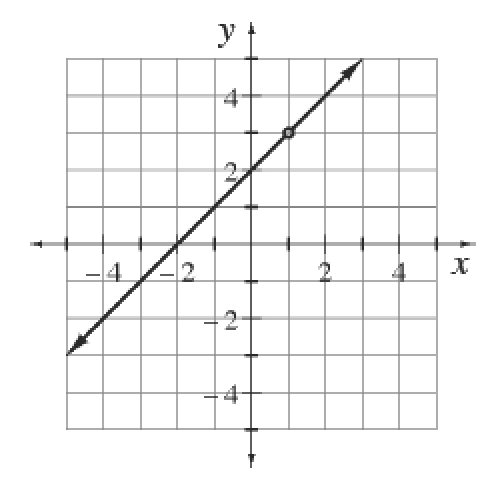### Home > CALC3RD > Chapter Ch1 > Lesson 1.2.3 > Problem1-51

1-51.

Analyze the graph of $y=\frac{(x+2)(x-1)}{x-1}$ at right.

1. What does $y$ approach as $x → ∞$? What does $y$ approach as $x→ −∞$? Describe how your answer can be predicted from the given equation.

The graph appears to be the linear function $y = x + 2$, with a hole at $(1,3)$.

Just like the graph of $y = x + 2$, this function will go up ($y →+∞$) as we move to the right ($x →∞$)

2. What does $y$ approach as $x → 1^−$ ($1$ from the left)? What does $y$ approach as $x → 1^+$ ($1$ from the right)? Describe how your answer can be predicted from the given equation.

Refer to the hint in part (a). As the graph approaches the hole from the left ($x → −1$), what $y$-value does it approach